# Elastic Stack 实现地理空间数据采集与可视化分析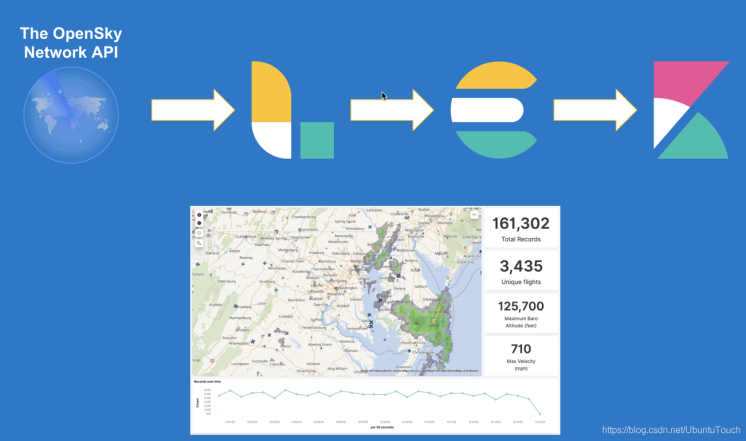### Logstash 配置文件

#### fligths_logstash.conf

input {
http_poller {
codec => "json"
schedule => { every => "15s" }
urls => {
url => "https://opensky-network.org/api/states/all"
}
}
}

filter {
split {
field => "states"
"icao" => "%{[states]}"
"callsign" => "%{[states]}"
"origin_country" => "%{[states]}"
"time_position" => "%{[states]}"
"last_contact" => "%{[states]}"
"location" => "%{[states]},%{[states]}"
"baro_altitude" => "%{[states]}"
"on_ground" => "%{[states]}"
"velocity" => "%{[states]}"
"true_track" => "%{[states]}"
"vertical_rate" => "%{[states]}"
"geo_altitude" => "%{[states]}"
"squawk" => "%{[states]}"
"spi" => "%{[states]}"
"position_source" => "%{[states]}"
}
}
mutate {
strip => ["callsign"]
rename => { "time" => "request_time" }
remove_field => ["states", "@version"]
}
translate {
field => "[position_source]"
destination => "position_source"
override => "true"
dictionary => {
"1" => "ASTERIX"
"2" => "MLAB"
}
}

if [time_position] =~ /^%{*/ {
drop { }
}
if [callsign] =~ /^%{*/ {
mutate { remove_field => ["callsign"] }
}
if [location] =~ /^%{*/ {
mutate { remove_field => ["location"] }
}
if [baro_altitude] =~ /^%{*/ {
mutate { remove_field => ["baro_altitude"] }
}
if [velocity] =~ /^%{*/ {
mutate { remove_field => ["velocity"] }
}
if [true_track] =~ /^%{*/ {
mutate { remove_field => ["true_track"] }
}
if [vertical_rate] =~ /^%{*/ {
mutate { remove_field => ["vertical_rate"] }
}
if [sensors] =~ /^%{*/ {
mutate { remove_field => ["sensors"] }
}
if [geo_altitude] =~ /^%{*/ {
mutate { remove_field => ["geo_altitude"] }
}
if [squawk] =~ /^%{*/ {
mutate { remove_field => ["squawk"] }
}

mutate {
convert => {
"baro_altitude" => "float"
"geo_altitude" => "float"
"last_contact" => "integer"
"on_ground" => "boolean"
"request_time" => "integer"
"spi" => "boolean"
"squawk" => "integer"
"time_position" => "integer"
"true_track" => "float"
"velocity" => "float"
"vertical_rate" => "float"
}
}
}

output {
stdout {
codec => rubydebug
}

elasticsearch {
manage_template => "false"
index => "flights"
# pipeline => "flights_aircraft_enrichment"
hosts => "localhost:9200"
}
}

input {
http_poller {
codec => "json"
schedule => { every => "15s" }
urls => {
url => "https://opensky-network.org/api/states/all"
}
}
}

PUT flights
{
"mappings": {
"properties": {
"@timestamp": {
"type": "date"
},
"baro_altitude": {
"type": "float"
},
"callsign": {
"type": "keyword"
},
"geo_altitude": {
"type": "float"
},
"icao": {
"type": "keyword"
},
"last_contact": {
"type": "long"
},
"location": {
"type": "geo_point"
},
"on_ground": {
"type": "boolean"
},
"origin_country": {
"type": "keyword"
},
"position_source": {
"type": "keyword"
},
"request_time": {
"type": "long"
},
"spi": {
"type": "boolean"
},
"squawk": {
"type": "long"
},
"time_position": {
"type": "long"
},
"true_track": {
"type": "float"
},
"velocity": {
"type": "float"
},
"vertical_rate": {
"type": "float"
}
}
}
}GET _cat/indices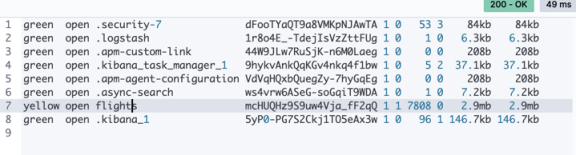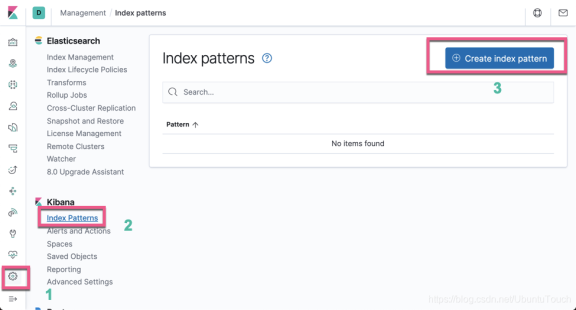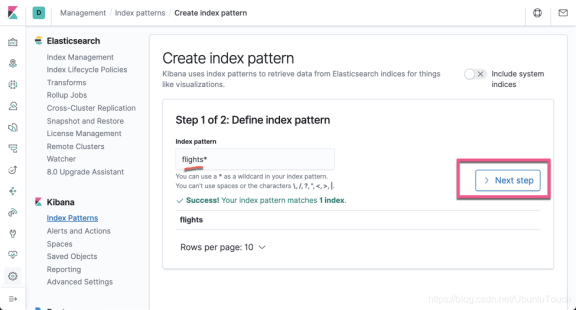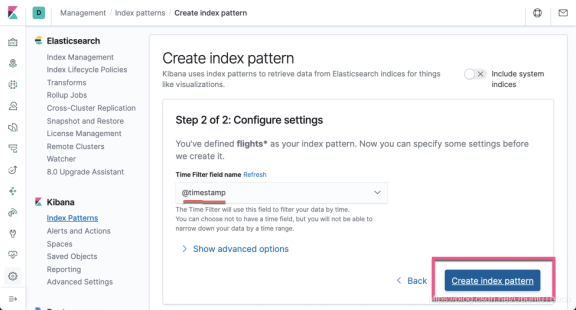### 运用 Kibana 可视化

#### 显示目前所有的正在飞行的飞机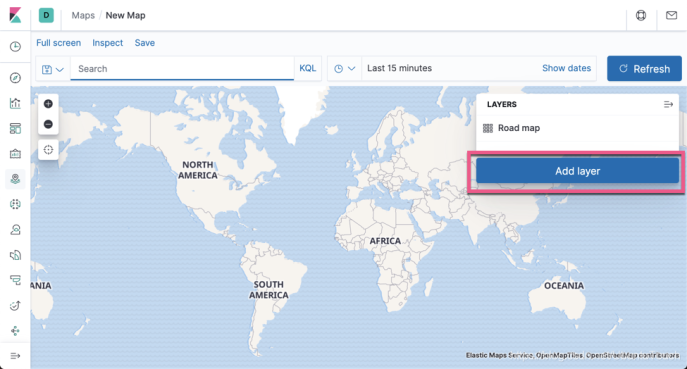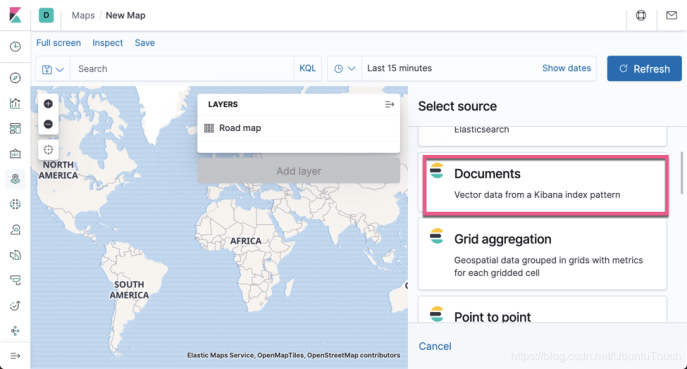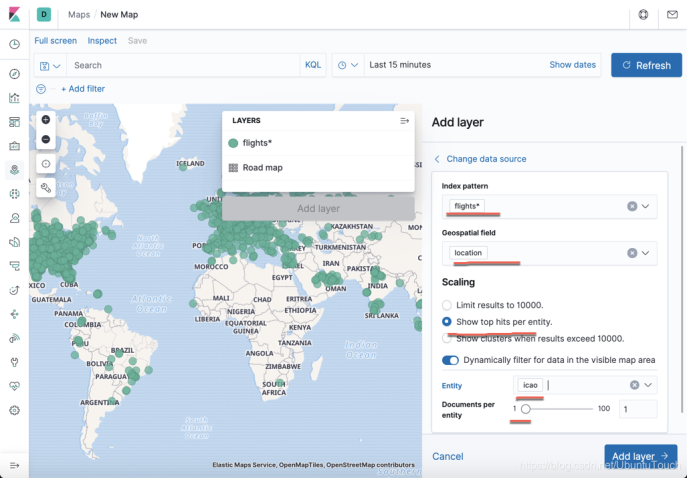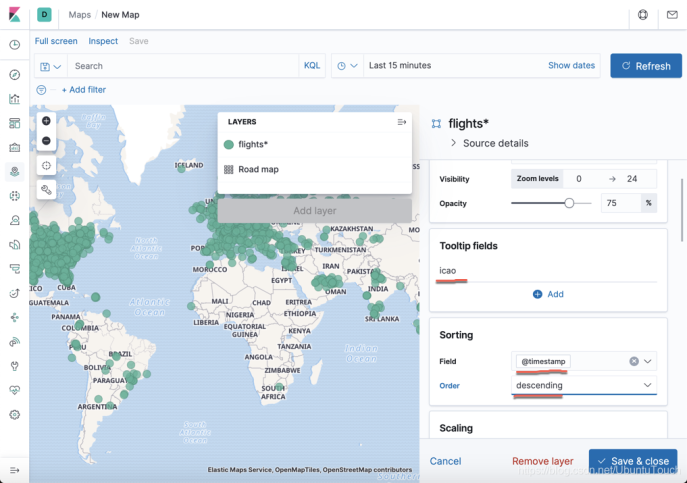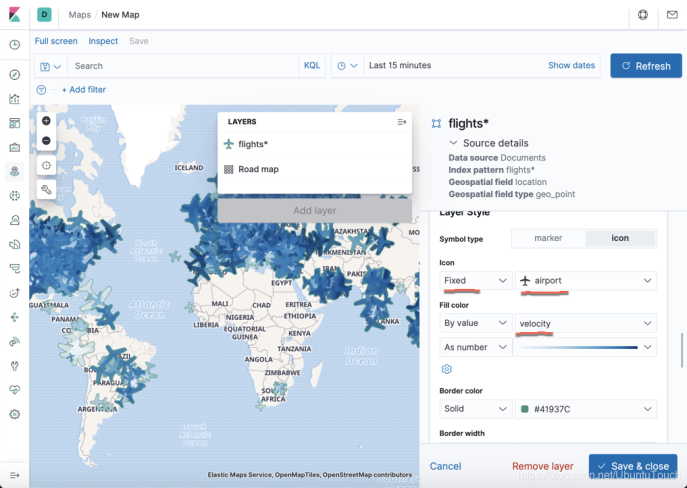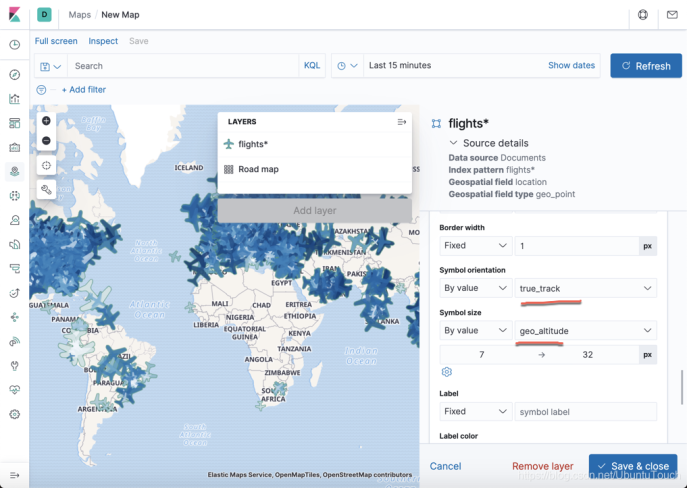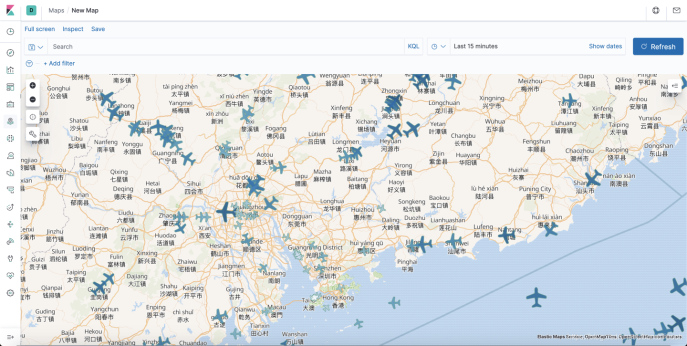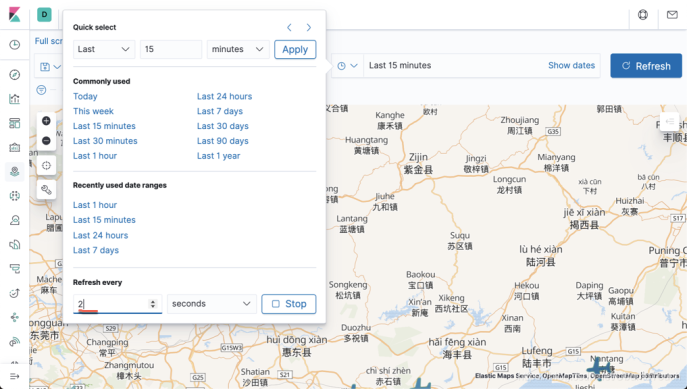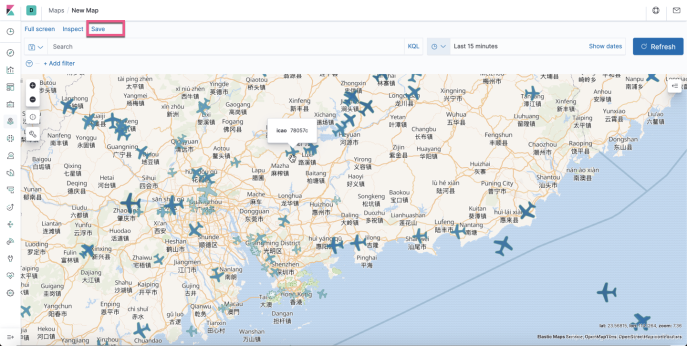### 显示飞机过去7天的数据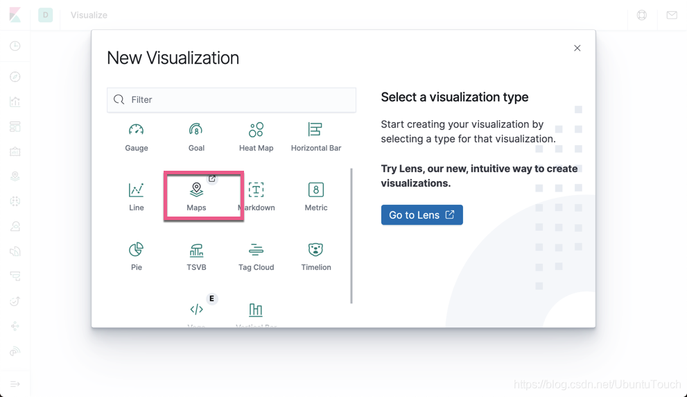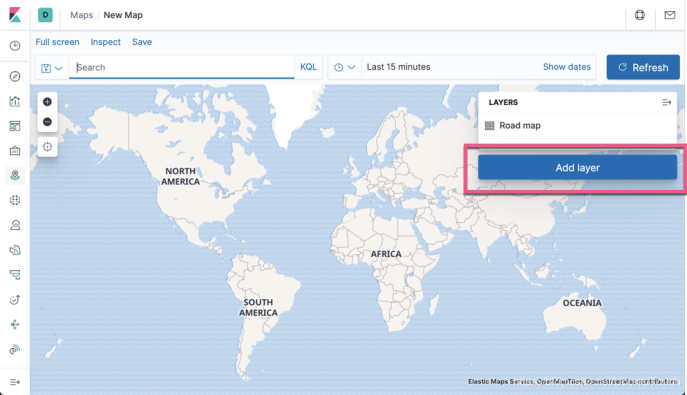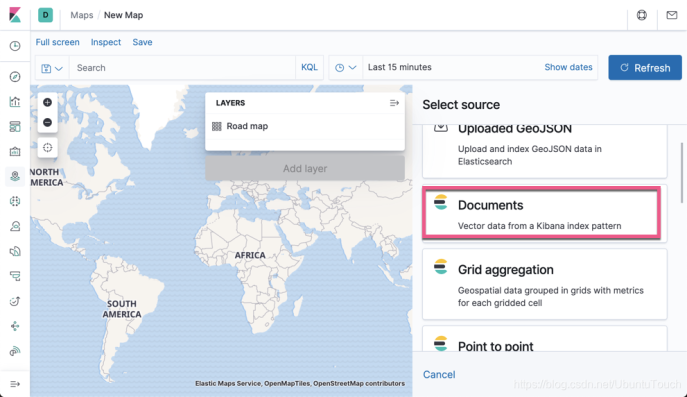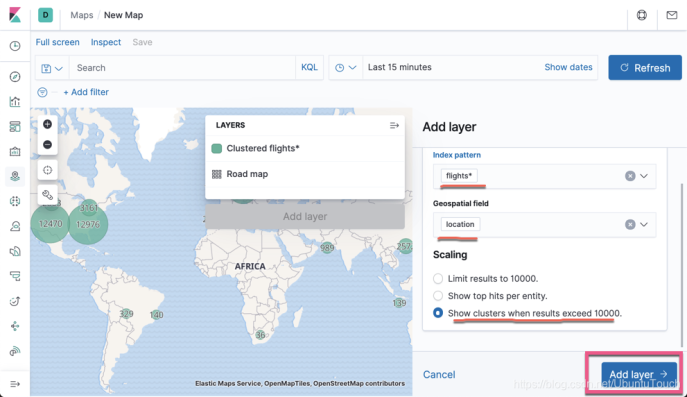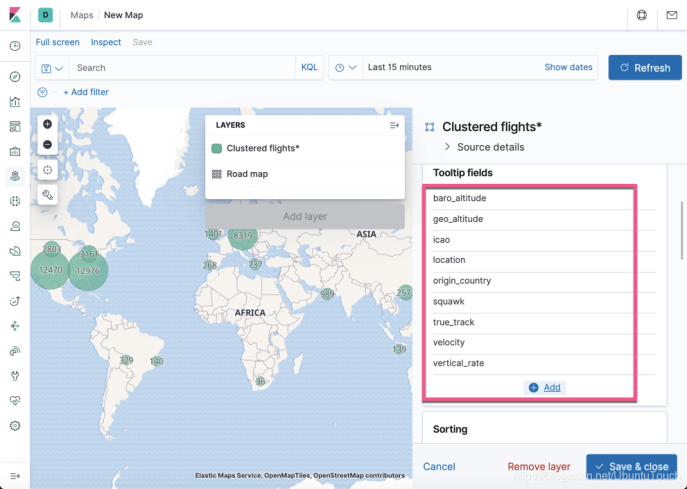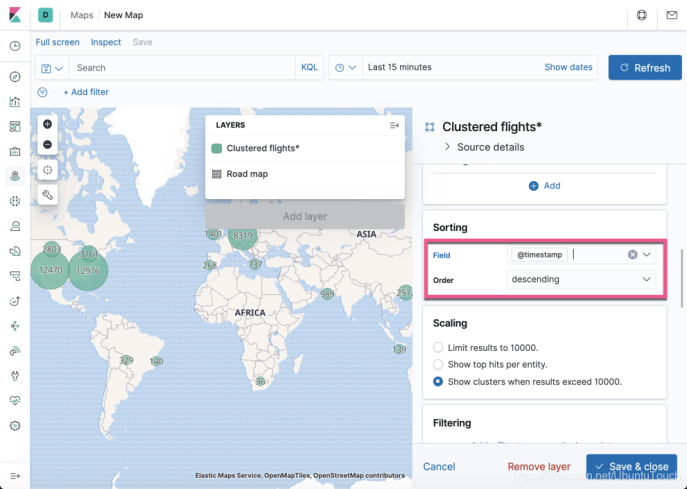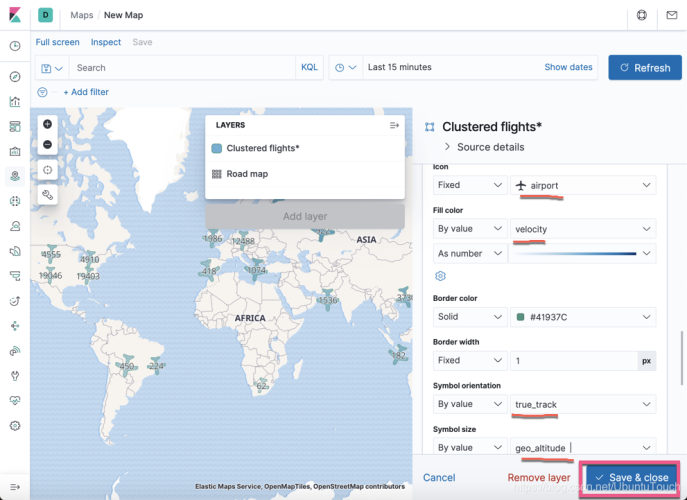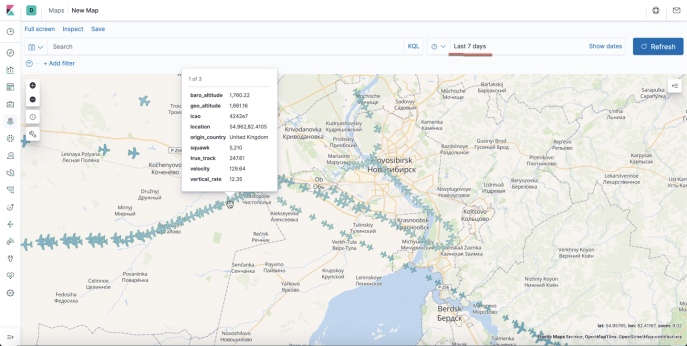### 查看哪个国家的飞机航班多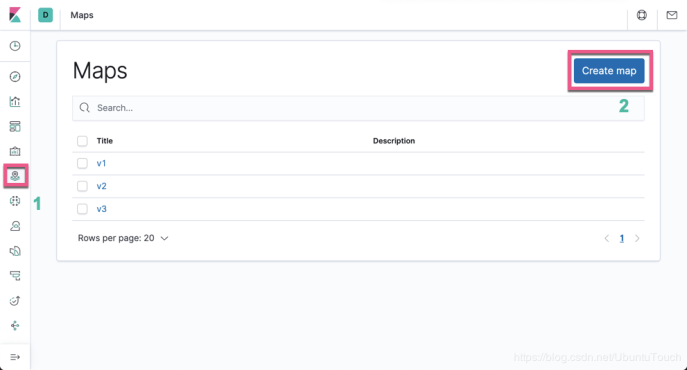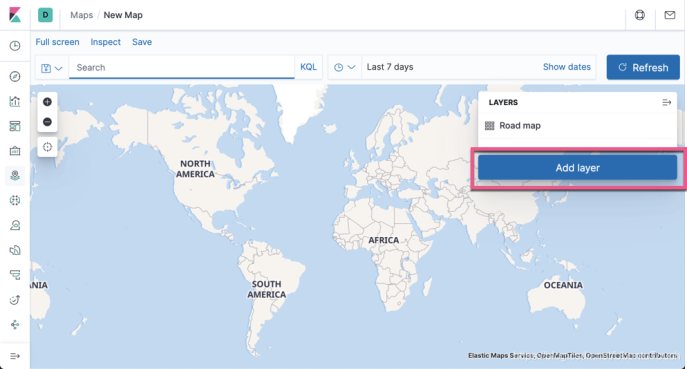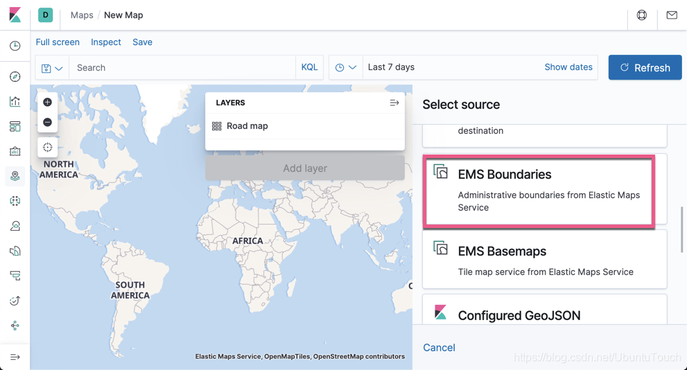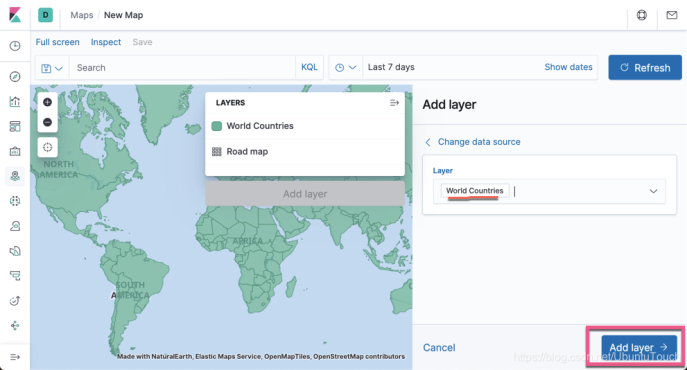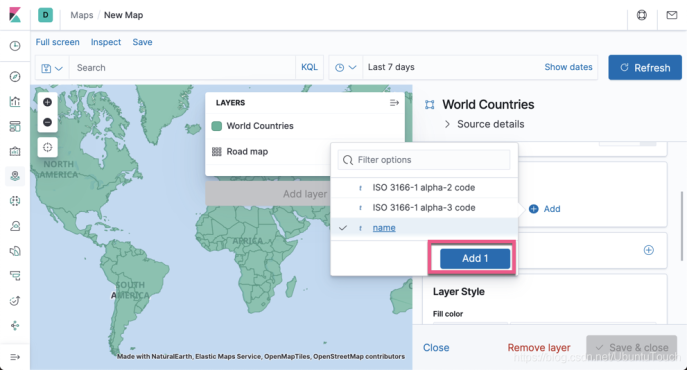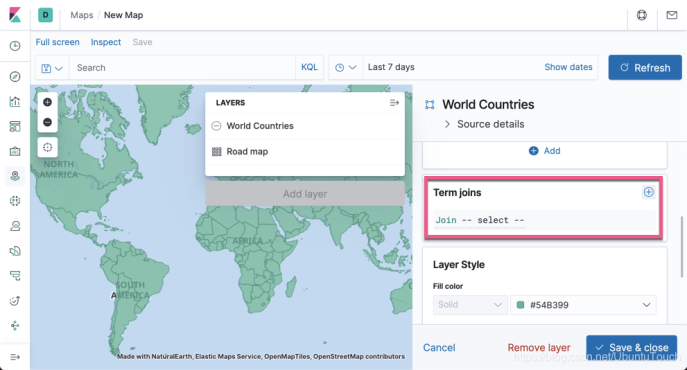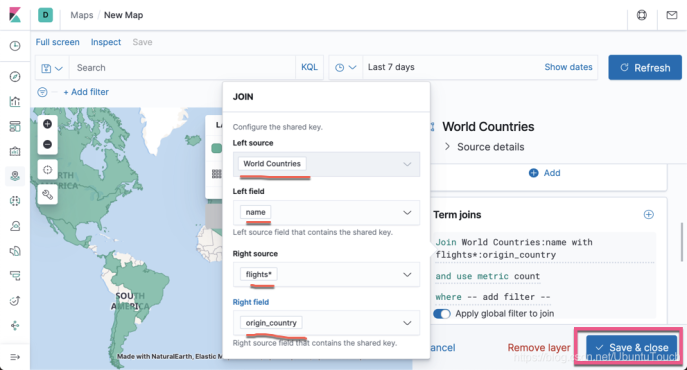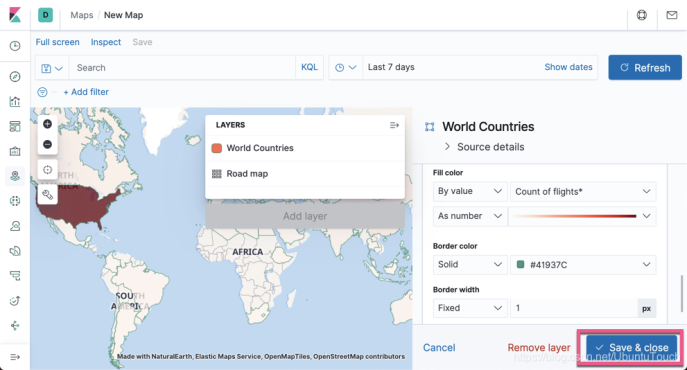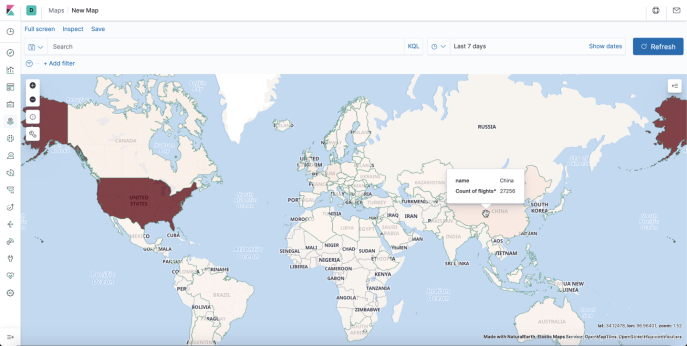### 找出各个地区的机场数目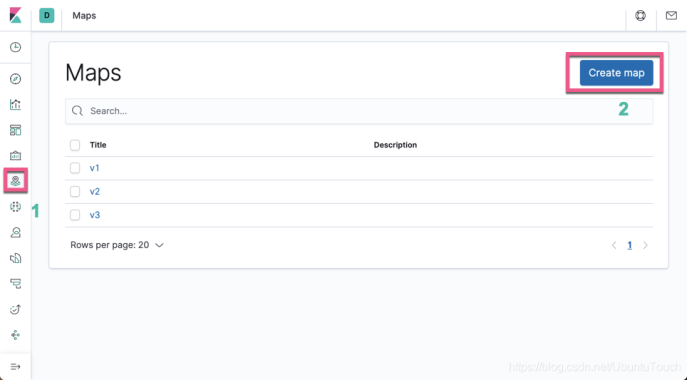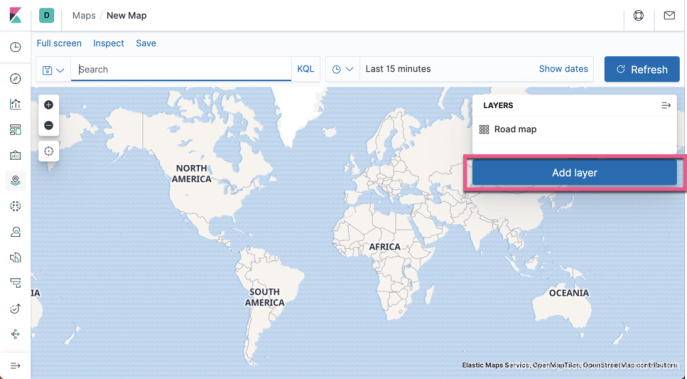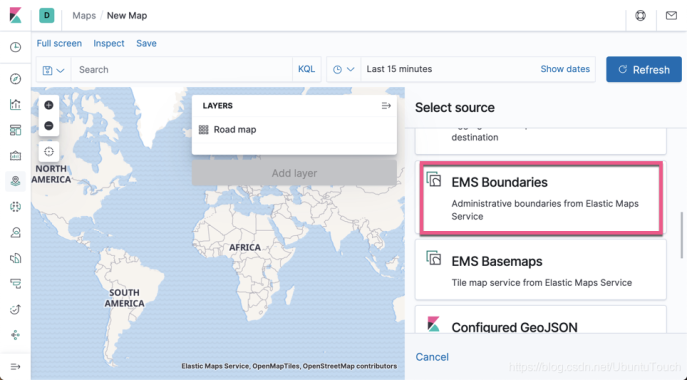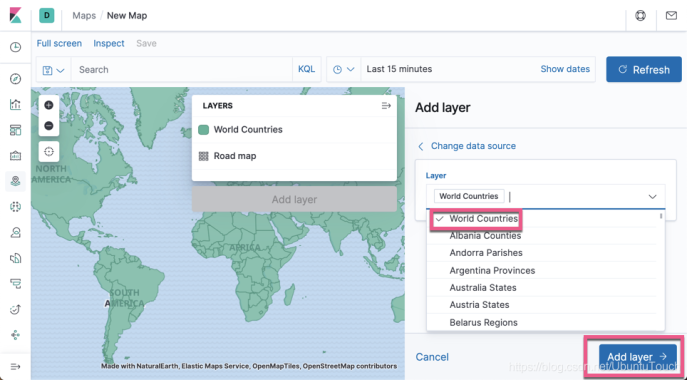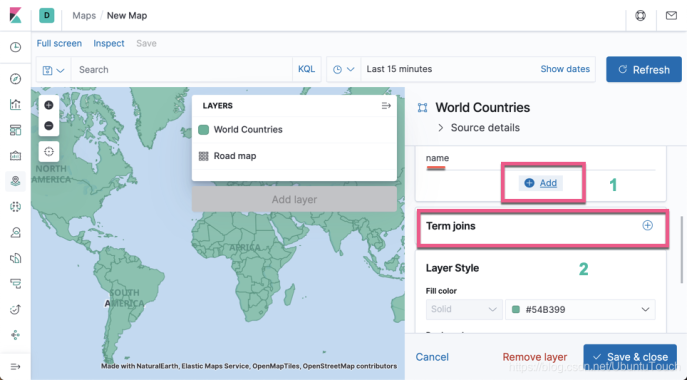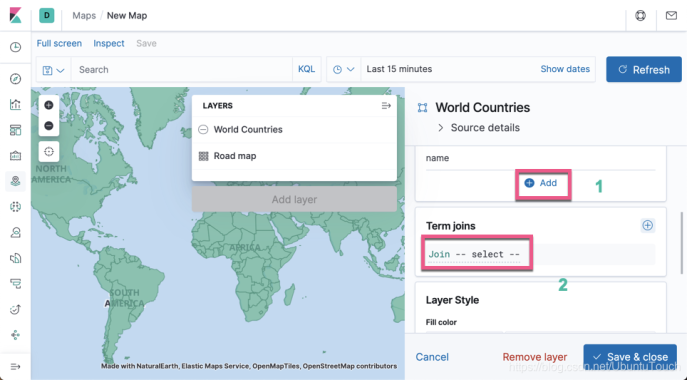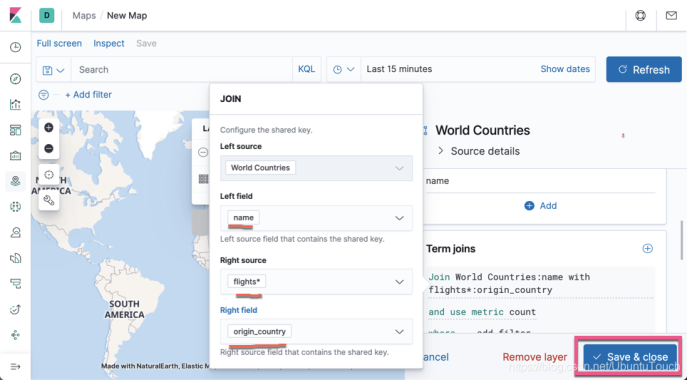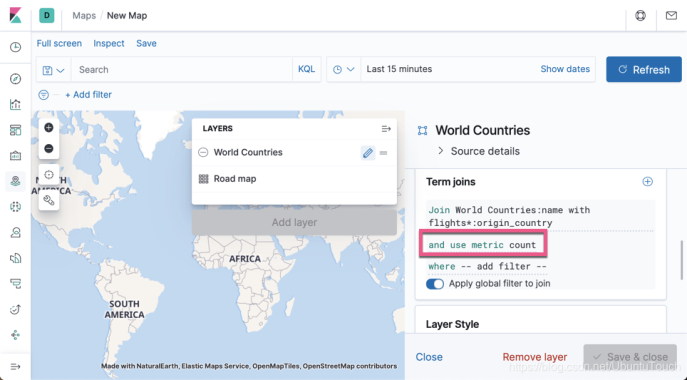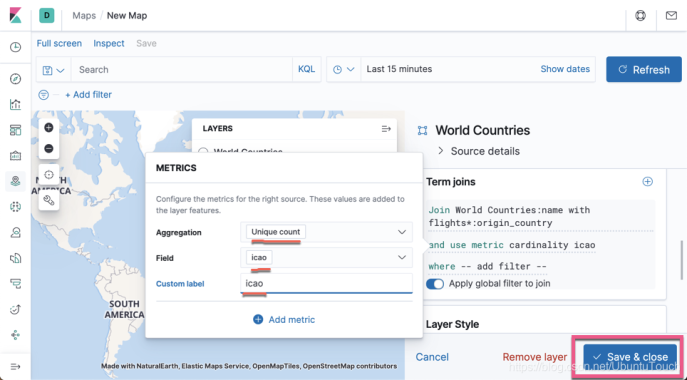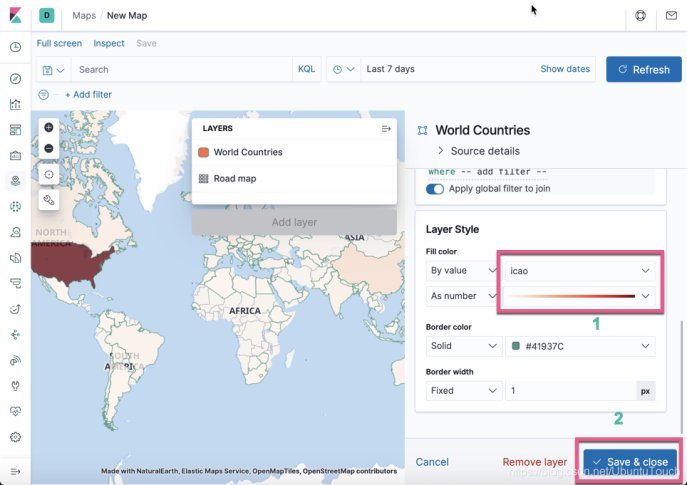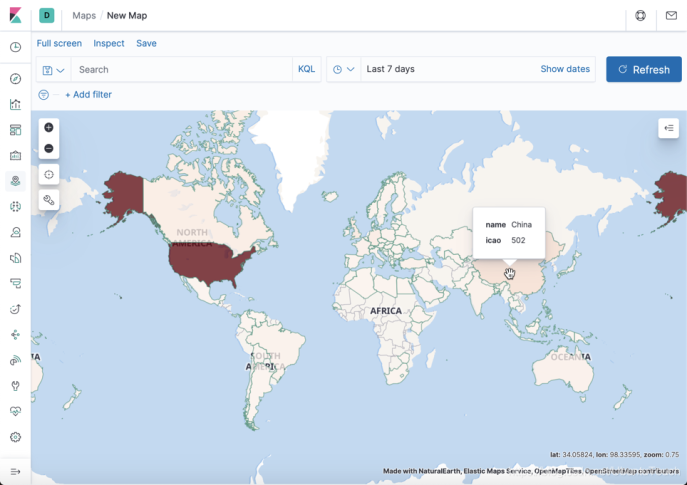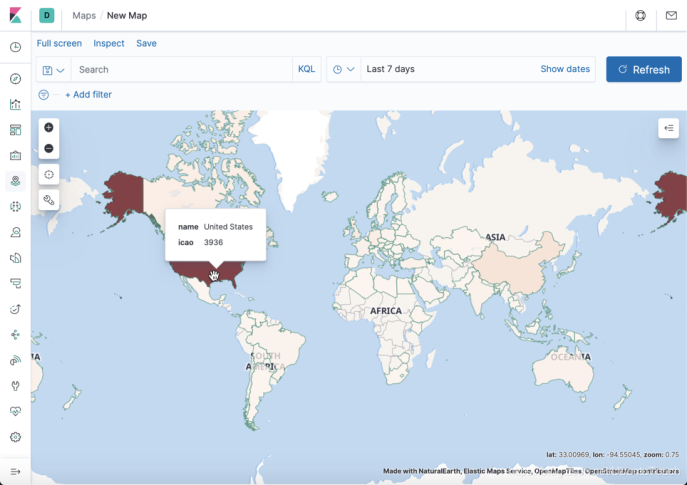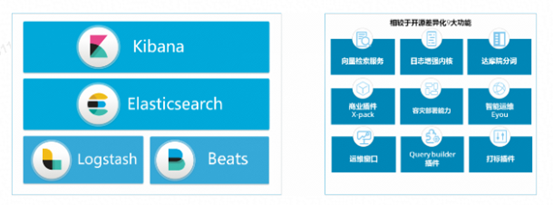### 相关活动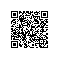Elasticsearch 作为一个分布式、高扩展、实时的搜索与数据分析引擎，因其轻量级、稳定、可靠、快速等特性受到越来越多开发者的青睐，在搜索、日志分析、运维监控和安全分析等领域得到广泛应用。